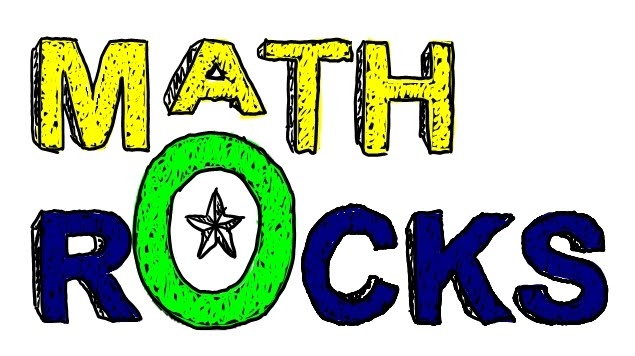•In Room 402 we will expand math concepts that have been learned in previous years and stretch our thinking as we tackle more complex math concepts.  We will work on learning multiple problem-solving methods and strengthen our mathematical stamina.  See below for a general overview of the topics covered in fifth grade.

Units of Study

Unit 1:    Number Sense/Operations (place value, multiplying and dividing multi-digit numbers)
Unit 2:    Fractions (unlike denominators, mixed numbers, multiplying and dividing)
Unit 3:    Decimals (multiplying and dividing)
Unit 4:    Geometry and Measurement (classifying shapes, finding volume for 3D shapes and conversions)
Unit 5:    Graphing and Data Analysis (reading and creating graphs)

Dreambox Learning

DreamBox is a tool we use to practice our math skills.  There are two options for accessing this tool.